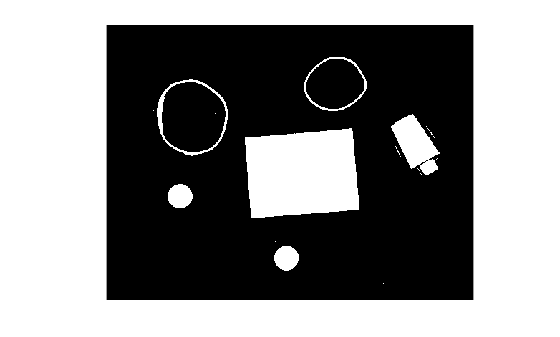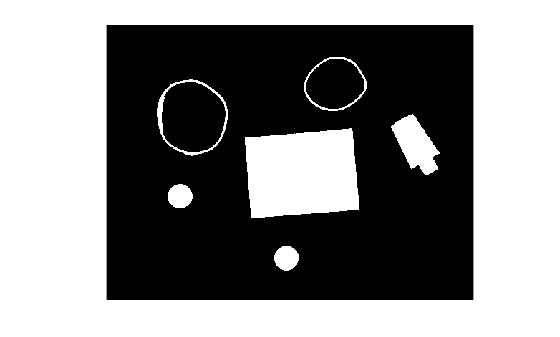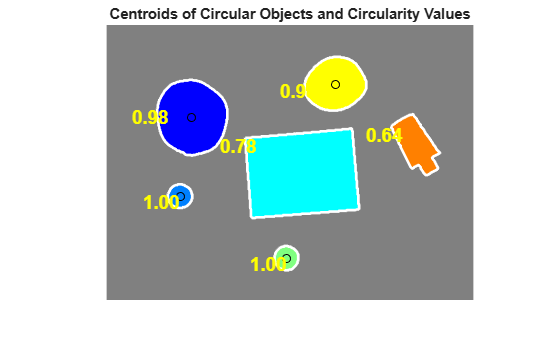Documentation

Identifying Round Objects

This example shows how to classify objects based on their roundness using bwboundaries, a boundary tracing routine.

Step 1: Read Image

imshow(RGB)Step 2: Threshold the Image

Convert the image to black and white in order to prepare for boundary tracing using bwboundaries.

I = rgb2gray(RGB);
bw = imbinarize(I);
imshow(bw)Step 3: Remove the Noise

Using morphology functions, remove pixels which do not belong to the objects of interest.

Remove all object containing fewer than 30 pixels.

bw = bwareaopen(bw,30);
imshow(bw)Fill a gap in the pen's cap.

se = strel('disk',2);
bw = imclose(bw,se);
imshow(bw)Fill any holes, so that regionprops can be used to estimate the area enclosed by each of the boundaries

bw = imfill(bw,'holes');
imshow(bw)Step 4: Find the Boundaries

Concentrate only on the exterior boundaries. Option 'noholes' will accelerate the processing by preventing bwboundaries from searching for inner contours.

[B,L] = bwboundaries(bw,'noholes');

Display the label matrix and draw each boundary.

imshow(label2rgb(L,@jet,[.5 .5 .5]))
hold on
for k = 1:length(B)
boundary = B{k};
plot(boundary(:,2),boundary(:,1),'w','LineWidth',2)
endStep 5: Determine which Objects are Round

Estimate each object's area and perimeter. Use these results to form a simple metric indicating the roundness of an object:

$\mathrm{metric}=\frac{4\pi *\mathrm{area}}{{\mathrm{perimeter}}^{2}}$

This metric is equal to 1 only for a circle and it is less than one for any other shape. The discrimination process can be controlled by setting an appropriate threshold. In this example use a threshold of 0.94 so that only the pills will be classified as round.

Use regionprops to obtain estimates of the area for all of the objects. Notice that the label matrix returned by bwboundaries can be reused by regionprops.

stats = regionprops(L,'Area','Centroid');

threshold = 0.94;

% loop over the boundaries
for k = 1:length(B)

% obtain (X,Y) boundary coordinates corresponding to label 'k'
boundary = B{k};

% compute a simple estimate of the object's perimeter
delta_sq = diff(boundary).^2;
perimeter = sum(sqrt(sum(delta_sq,2)));

% obtain the area calculation corresponding to label 'k'
area = stats(k).Area;

% compute the roundness metric
metric = 4*pi*area/perimeter^2;

% display the results
metric_string = sprintf('%2.2f',metric);

% mark objects above the threshold with a black circle
if metric > threshold
centroid = stats(k).Centroid;
plot(centroid(1),centroid(2),'ko');
end

text(boundary(1,2)-35,boundary(1,1)+13,metric_string,'Color','y',...
'FontSize',14,'FontWeight','bold')

end

title(['Metrics closer to 1 indicate that ',...
'the object is approximately round'])